NEET  >  Irodov Solutions: Kinetic Theory of Gases - 1

# Irodov Solutions: Kinetic Theory of Gases - 1 - Notes | Study Physics Class 11 - NEET

 1 Crore+ students have signed up on EduRev. Have you?

Q. 62. Modern vacuum pumps permit the pressures down to p = 4.10-15  atm to be reached at room temperatures. Assuming that the gas exhausted is nitrogen, find the number of its molecules per 1 cm3  and the mean distance between them at this pressure.

Solution. 62. From the formula p - n k T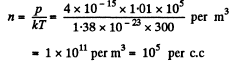Mean distance between molecules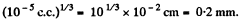Q. 63. A vessel of volume V = 5.0 l contains m = 1.4 g of nitrogen at a temperature T = 1800 K. Find the gas pressure, taking into account that η = 30% of molecules are disassociated into atoms at this temperature.

Solution. 63. A fter dissociation each N2 molecule becomes two Adatoms and so contributes, 2 x 3 degrees of freedom. Thus the number of moles becomes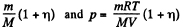Here M is the molecular weight in grams of N2.

Q. 64. Under standard conditions the density of the helium and nitrogen mixture equals p = 0.60 g/l. Find the concentration of helium atoms in the given mixture.

Solution. 64. Let n1 = number density of He atoms, n2 = number density of N2 molecules

Then p = n1 m1 + n2 m2

where m1 = mass of He atom, m2 = mass of N2 molecule also p = (n1 + n2) kT

From these two equations we get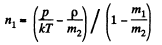Q. 65. A parallel beam of nitrogen molecules moving with velocity v = 400 m/s impinges on a wall at an angle θ = 30° to its normal. The concentration of molecules in the beam n = 0.9.1019  cm-3. Find the pressure exerted by the beam on the wall assuming the molecules to scatter in accordance with the perfectly elastic collision law.

Solution. 65.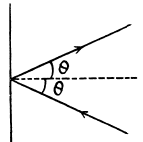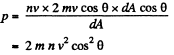Q. 66. How many degrees of freedom have the gas molecules, if under standard conditions the gas density is p = 1.3 mg/cm3 and the velocity of sound propagation in it is v = 330 m/s.

Solution. 66. From the formula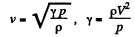If i = number of degrees of freedom of the gas then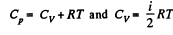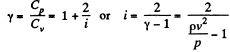Q. 67. Determine the ratio of the sonic velocity v in a gas to the root mean square velocity of molecules of this gas, if the molecules are
(a) monatomic; (b) rigid diatomic.

Solution. 67.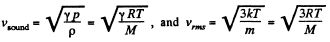so,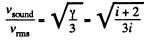(a) For monoatomic gases i = 3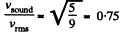(b) For rigid diatomic molecules i = 5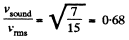Q. 68. A gas consisting of N-atomic molecules has the temperature T at which all degrees of freedom (translational, rotational, and vibrational) are excited. Find the mean energy of molecules in such a gas. What fraction of this energy corresponds to that of translational motion?

Solution. 68. For a general noncollinear, nonplanar molecule

mean energy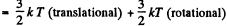+ (3N - 6) kT (vibrational)

= (3N - 3) kT per molecule

For linear molecules, mean energy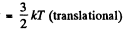+ kT (rotational) + (3 N - 5) kT (vibrational)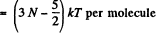Translational energy is a fraction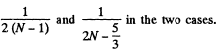Q. 69. Suppose a gas is heated up to a temperature at which all degrees of freedom (translational, rotational, and vibrational) of its molecules are excited. Find the molar heat capacity of such a gas in the isochoric process, as well as the adiabatic exponent γ, if the gas consists of
(a) diatomic;
(b) linear N-atomic;
(c) network N-atomic molecules.

Solution. 69. (a) A diatomic molecule has 2 translational, 2 rotational and one vibrational degrees of freedom. The corresponding energy per mole is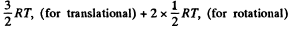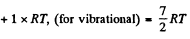Thus,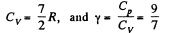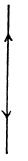(b) For linear N - atomic molecules energy per mole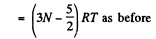So,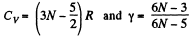(c) For noncollinear N- atomic molecules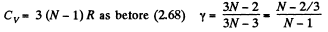Q. 70. An ideal gas consisting of N-atomic molecules is expanded isobarically. Assuming that all degrees of freedom (translational, rotational, and vibrational) of the molecules are excited, find what fraction of heat transferred to the gas in this process is spent to perform the work of expansion. How high is this fraction in the case of a monatomic gas?

Solution. 70. In the isobaric process, work done is

A = pdv = RdT per mole.

On the other hand heat transferred Q = CpdT

Now Cp = (3N - 2) R for non-collinear molecules and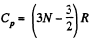for linear molecules

ThusFor monoatomic gases,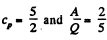Q. 71. Find the molar mass and the number of degrees of freedom of molecules in a gas if its heat capacities are known: cv = 0.65 J/(g•K) and cp  = 0.91 J/(g•K).

Solution. 71. Given specific heats cp, cv (per unit mass)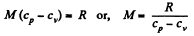Also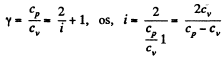Q. 72. Find the number of degrees of freedom of molecules in a gas whose molar heat capacity
(a) at constant pressure is equal to Cp  = 29 J/(mol.K);
(b) is equal to C = 29 J/(mol•K) in the process pT = const.

Solution. 72.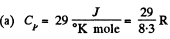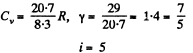(b) In the process pT = const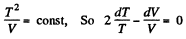Thus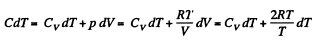or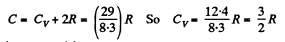Hence i = 3 (monoatomic)

Q. 73. Find the adiabatic exponent γ for a mixture consisting of v1 moles of a monatomic gas and v2 moles of gas of rigid diatomic molecules.

Solution. 73.  Obviously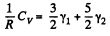(Since a monoatomic gas has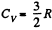and a diatomic gas has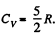[The diatomic molecule is rigid so no vibration])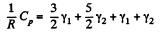Hence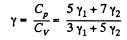Q. 74. A thermally insulated vessel with gaseous nitrogen at a temperature t = 27°C moves with velocity v = 100 m/s. How much (in per cent) and in what way will the gas pressure change on a sudden stoppage of the vessel?

Solution. 74. The internal energy of the molecules are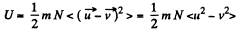where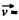velocity of the vessel, N = number of molecules, each of mass m. When the vessel is stopped, internal energy becomes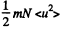So there is an increase in internal energy of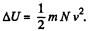This will give rise to a rise in temperature of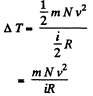there being no flow of heat This change of temperature will lead to an excess pressure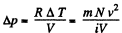and finally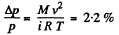where M = molecular weight of N2 , i = number of degrees of freedom of N2

Q. 75. Calculate at the temperature t = 17°C:
(a) the root mean square velocity and the mean kinetic energy of an oxygen molecule in the process of translational motion;
(b) the root mean square velocity of a water droplet of diameter d = 0.10 μm suspended in the air.

Solution. 75. (a) From the equipartition theorem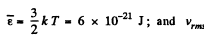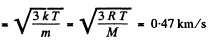(b) In equilibrium the mean kinetic energy of the droplet will be equal to that of a molecule.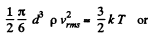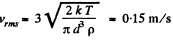Q. 76. A gas consisting of rigid diatomic molecules is expanded adiabatically. How many times has the gas to be expanded to reduce the root mean square velocity of the molecules η = 1.50 times?

Solution. 76.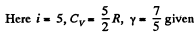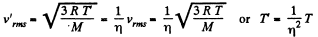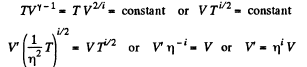The gas must be expanded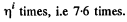Q. 77. The mass m = 15 g of nitrogen is enclosed in a vessel at a temperature T = 300 K. What amount of heat has to be transferred to the gas to increase the root mean square velocity of its molecules η = 2.0 times?

Solution. 77. Here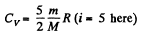m = mass of the gas, M = molecular weight If vrms increases η times, the temperature will have increased η2 times. This will require (neglecting expansion of the vessels) a heat flow of amount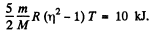Q. 78. The temperature of a gas consisting of rigid diatomic molecules is T = 300 K. Calculate the angular root mean square velocity of a rotating molecule if its moment of inertia is equal to I = 2.1.10-39  g• cm2.

Solution. 78. The root mean square angular velocity is given by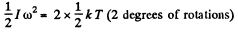or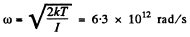Q. 79. A gas consisting of rigid diatomic molecules was initially under standard conditions. Then the gas was compressed adiabatically η = 5.0 times. Find the mean kinetic energy of a rotating molecule in the final state.

Solution. 79. Under compression, the temperature will rise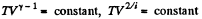or,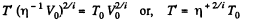Q. 80. How will the rate of collisions of rigid diatomic molecules against the vessel's wall change, if the gas is expanded adiabatically η times?

Solution. 80.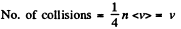Now,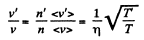(When the gas is expanded η times, n decreases by a factor η). Also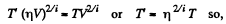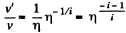i.e. collisions decrease by a factor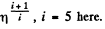Q. 81. The volume of gas consisting of rigid diatomic molecules was increased η = 2.0 times in a polytropic process with the molar heat capacity C = R. How many times will the rate of collisions of molecules against a vessel's wall be reduced as a result of this process?

Solution. 81. In a polytropic process pVn = constant., where n is called the polytropic index. For this process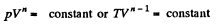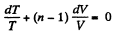Then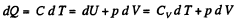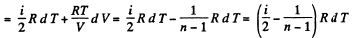Now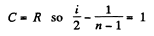or,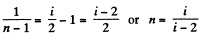Now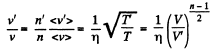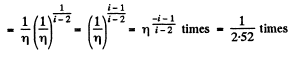Q. 82. A gas consisting of rigid diatomic molecules was expanded in a polytropic process so that the rate of collisions of the molecules against the vessel's wall did not change. Find the molar heat capacity of the gas in this process.

Solution. 82. If a is the polytropic index then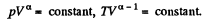Now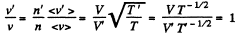Hence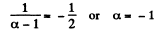Then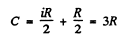The document Irodov Solutions: Kinetic Theory of Gases - 1 - Notes | Study Physics Class 11 - NEET is a part of the NEET Course Physics Class 11.
All you need of NEET at this link: NEET

## Physics Class 11

127 videos|464 docs|210 tests

## Physics Class 11

127 videos|464 docs|210 tests

### How to Prepare for NEET

Read our guide to prepare for NEET which is created by Toppers & the best Teachers

Track your progress, build streaks, highlight & save important lessons and more!

,

,

,

,

,

,

,

,

,

,

,

,

,

,

,

,

,

,

,

,

,

;